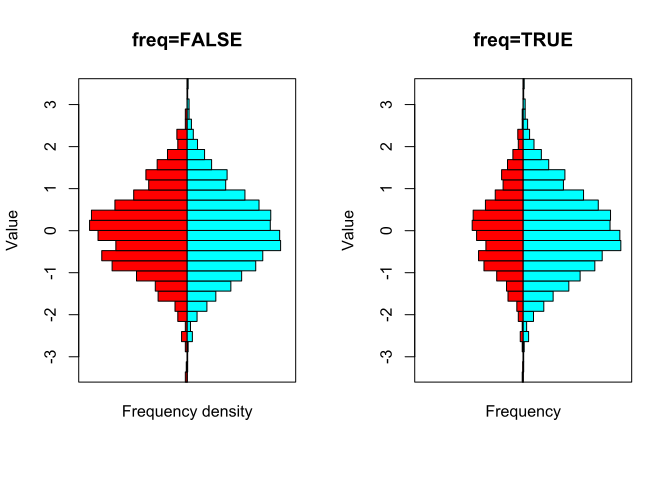# xyloplot examples

#### 2018-05-09

The xyloplot function displays continuous or discrete data as histogram style violin plots, or “xylophones”.

xyloplot accepts a simple numeric vector…

xyloplot(rnorm(1000))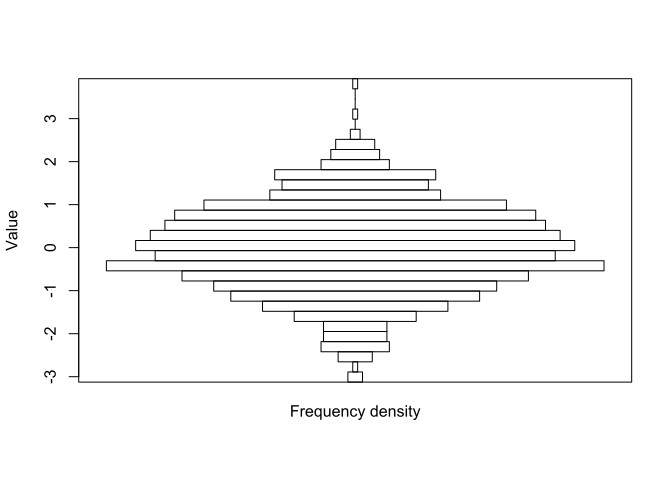… or a list of simple numeric vectors…

xyloplot(
x=lapply(1:3, function(mean) rnorm(mean=mean, n=1000)),
breaks=20,
col=rainbow(3),
main="title")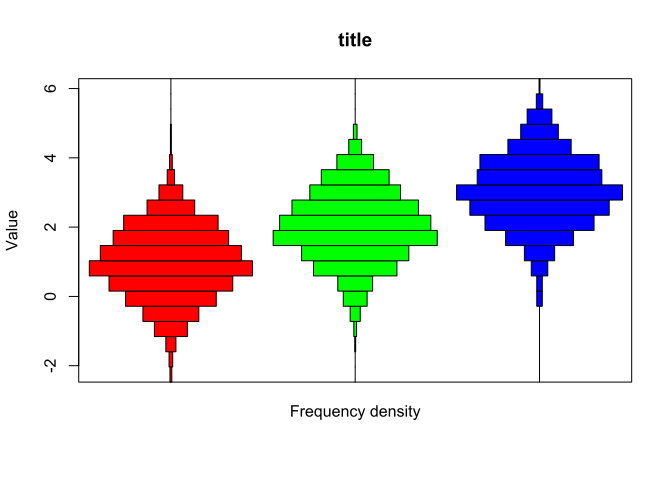… or discrete numeric data (supplied as a factor)…

xyloplot(
replicate(n=5, simplify=FALSE,
expr=factor(sample(c(0.01, 0.1, 0.2, 0.25, 0.5, 1), size=10, replace=TRUE))),
col=rainbow(5))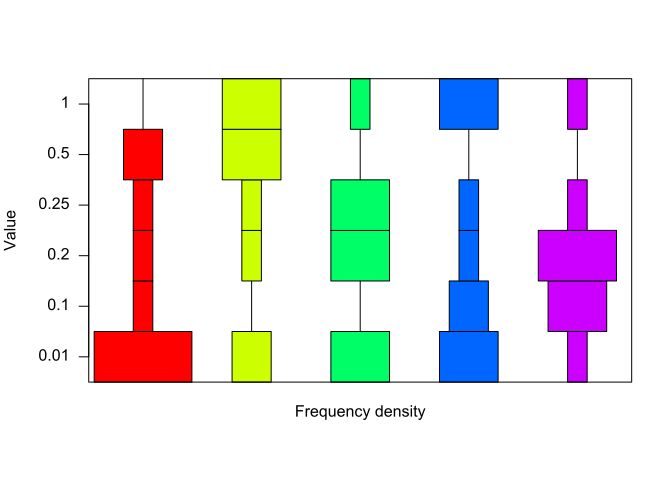… or general factors with wordy levels…

xyloplot(
sample(c("goldfish","cat","dog","fish","mouse","giraffe"),
size=100, replace=TRUE))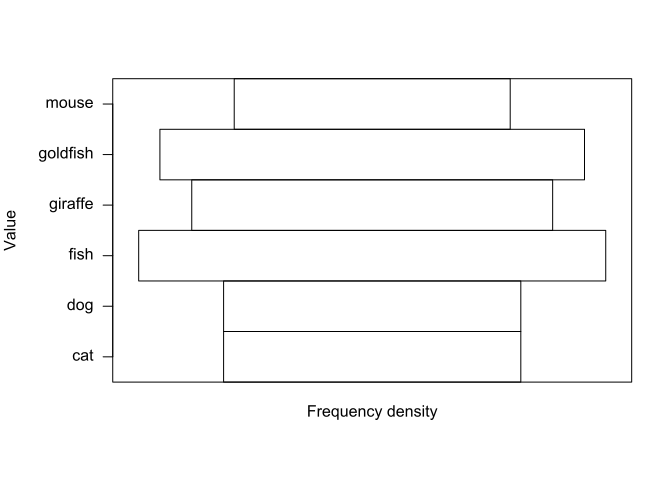The xylo_positions function gives you the x-coordinates of the centres of the xylophones in case you want to add further graphical objects. For example, here we’ll add the upper quartile as a red line for samples from 3 normal distributions.

data <- lapply(1:3, function(mean) rnorm(mean=mean, n=1000))
xyloplot(x=data)
n <- length(data)
positions <- xylo_positions(n)
upper_qs <- sapply(data, function(sample) quantile(sample, prob=0.75))
segments(x0=positions-1/n/2, x1=positions+1/n/2, y0=upper_qs, y1=upper_qs, col="red", pch=19)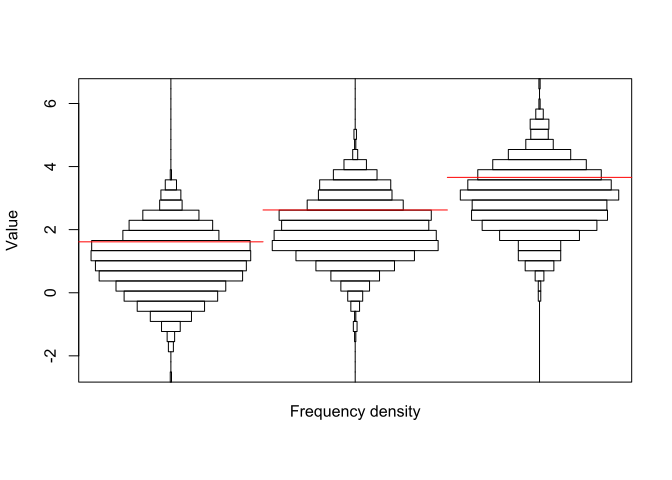xyloplot accepts an rhs argument, causing the xylophones to split in the middle, and plotting left hand side distributions x and right hand side ones from rhs. Note that graphical options, e.g. col, are recycled over the distributions going from left to right, regardless of whether they are lhs or rhs.

data_rhs <- lapply(2:4, function(mean) rnorm(mean=mean, sd=2, n=2000))
xyloplot(x=data, rhs=data_rhs, col=rainbow(2))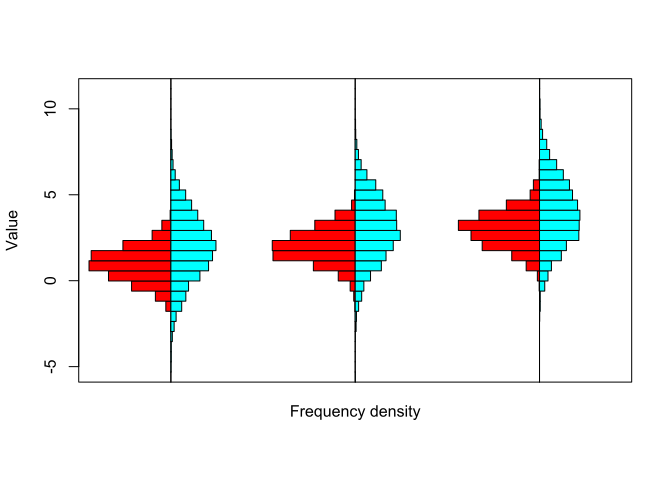xyloplot accepts a freq argument. It is equivalent to the freq argument in hist: a logical value which if FALSE (default) makes the width of bars represent frequency densities, and if TRUE, makes the width of bars represent frequencies/counts. In the example below, note that the rhs object contains twice as many observations as the lhs object. Setting freq to TRUE will therefore mean that the right-hand side bars collectively occupy twice as much width as the left-hand side ones.

lhs <- rnorm(n=1000)
rhs <- rnorm(n=2000)
par(mfrow=c(1, 2))
xyloplot(main="freq=FALSE", x=lhs, rhs=rhs, col=rainbow(2), freq=FALSE)
xyloplot(main="freq=TRUE", x=lhs, rhs=rhs, col=rainbow(2), freq=TRUE)